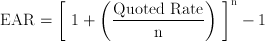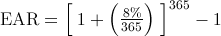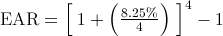# Business and Finance Math #2: Calculating the Effective Annual Rate (EAR) on Your TI BA II Plus or HP 12c

Filed under Difficulty: Easy, Finance, HP 12c, TI BA II Plus.

Consider the following problem:

Timmy Burns plans to open a new bank account and calls several banks to find out where he can earn the most interest on his money. After talking with several banks, Timmy has three options. Which bank account should he choose to earn the highest return on his money?

8% compounded daily
8.25% compounded quarterly
8.4% compounded annually

It’s not immediately obvious looking at these options which bank account Timmy should choose. At first glance, 8.4% compounded yearly seems to be the highest rate, but is it necessarily the best choice? Read on to learn about the mathematical theory to solve this problem, or skip down to see how you can quickly find the answer with your financial calculator.

## Effective Annual Rate (EAR): Mathematical Background

The key to solving this problem is understanding the relationship between the interest rate and rate of compounding. While Timmy is quoted several different interest rates, the rate he will actually earn on his bank account in each case also depends on the number of times per year interest is compounded on each bank account. To answer this question we need to determine and compare the effective annual rate (the interest rate that Timmy actually earns) for each bank account option.

Let’s start with a simple example: If you deposit \$1000 in a bank account paying 10% interest annually, after one year you would have \$1,100 in the bank account [\$1000 x (1 + .1)1] assuming no additional deposits or withdrawals.

If you deposit \$1,000 in a bank account paying 10% interest compounded semiannually, this means you earn 5% every six months (two times per year) on your bank account. After one year, you would have a bank account balance of \$1000 x (1 + .05)2 = \$1,102.50. Notice that this is \$2.50 higher than the bank account with 10% compounded once a year.

The reason for the additional \$2.50 in the bank account after one year is a result of the number of times your money is compounded per year (two times instead of only once). After the first six months, your bank account balance is \$1,050 [\$1000 x (1 + .05)1], with \$50 in interest earned on the \$1,000 deposit. At the end of the second six month period, you earn interest on the interest already earned during the first six months: [\$1,050 x (1.05)1 = \$1,102.50]. The \$2.50 is the compound interest (interest earned on interest) earned on the \$50 [\$50 x (1.05)1] in interest earned during the first six months.

Clearly, you would choose the bank account paying 10% semiannually because you would have more money in the account after one year than the bank account paying 10% compounded annually. While 10% quoted semiannually is the stated interest rate on the bank account (also known as the quoted interest rate or nominal rate), you actually earn 10.25% per year on an account paying 10% semiannually. The 10.25% interest rate is the effective annual rate, the rate you truly earn on your money over one year.

Now that we have calculated the effective annual interest rate, it is a no-brainer: you are better off choosing a bank account paying 10% compounded semiannually rather than a bank account paying 10% once per year.

Notice that by finding the effective annual rate of 10.25% for the account option paying 10% compounded semiannually, we now have an apples to apples comparison to the 10% compounded annually. Both interest rates are now expressed in terms of the same period of time, which makes the comparison simple: 10.25% per year is higher than 10% per year.

To quickly review, we just compared 10% compounded annually to 10% compounded semiannually. We can conclude:

• The more times per year your money is compounded, the higher the ending balance. This is because we are earning interest on interest already earned. Assuming you deposit \$1,000 in a bank account:

•  Interest Rate # Compoundings per Year Ending Value 10% compounded annually 1 \$1,100 10% compounded semiannually 2 \$1,102.50 10% compounded quarterly 4 \$1,103.81 10% compounded daily 365 \$1,105.16

• An interest rate quoted at 10% compounded semiannually is really the same thing as 10.25% compounded annually. The 10.25% interest rate is the effective interest rate, the rate you actually earn on your money
• The effective annual rate allows us to consistently compare different interest rates

Based on our example, we can derive a formula to convert a stated interest rate to the effective annual rate:In this equation, n is the number of compoundings per year. To produce this equation, we:

• Divide the quoted or stated interest rate by the number of times the interest is compounded per year (n)
• We then add one to this result, and raise this result to the power of the number of times the interest is compounded per year
• Finally, we subtract one and are left with the effective annual rate

## Calculating and Comparing the Effective Annual Rate (EAR)

Returning to Timmy’s choices, we can solve for the EAR in each case and compare the results:

8% Compounded daily:EAR = 8.33%

8% Compounded quarterly:EAR = 8.51%

 Interest Rate # Compoundings per Year EAR 8% compounded daily 365 8.33% 8.25% compounded quarterly 4 8.51% 8.4% compounded annually 1 8.4%

Based on our calculations, Timmy should open a bank account paying 8.25% interest compounded quarterly to earn the highest effective interest rate on his money (8.51%).

Now let’s see how to quickly solve this problem on our financial calculator. Note that for the TI-83 Plus and TI-84 Plus version of these calculations, check out our article on EAR on these graphing calculators.

## TI BA II Plus Version

### General Process to Calculate EAR on the TI BA II Plus

1. Press 2nd .This selects the ICONV function on the TI BA II Plus.
2. You should see “NOM=” on your calculator screen.
3. Enter the interest rate you want to convert to the EAR, then press ENTER
4. Press the button twice. You should see “C/Y=” on your calculator screen. On this screen you input the number of times per year your interest is compounded. Enter the interest rate, then press .
5. Finally, press the once. You should see “EFF=” on your calculator screen, which stands for the effective annual rate. Press the key and the EAR will be displayed on the calculator display.

Applying these steps to Timmy’s problem:

8% compounded daily

1. Press 2nd . Make sure the screen says “NOM=”
2. Press , then
3. Press the arrow twice, then type in 365 followed by
4. Press the once, then
5. The EAR should equal 8.33%

8.25% compounded quarterly

1. Press 2nd . Make sure the screen says “NOM=”
2. Input 8.25, then
3. Press the arrow twice, then type in 4 followed by
4. Press the once, then
5. The EAR should equal 8.51%

8.4% compounded annually is already an effective rate, so choosing from our calculations, 8.25% compounded quarterly is the best option for Timmy.

## HP 12c Version

The process for converting a nominal rate to an effective rate is slightly more complicated compared to the TI BA II Plus. We are going to demonstrate how to solve for the EAR using Reverse Polish Notation (RPN).

### General Process to Calculate EAR on the HP 12c

1. Make sure your HP 12c is set to Reverse Polish Notation (RPN) mode. If you see “RPN” on your calculator display you are ready for Step 2, otherwise activate RPN by pressing f
2. Make sure the calculator is set to “end” mode by pressing g
3. Clear out the financial registers by pressing f
4. Now you can convert the nominal rate to the effective rate. Enter the nominal rate into you calculator then press
5. The next step is to input the number of times per year compounding occurs. Enter the number of times compounded per year,, then
6. Key in 100, , followed by
7. Press , , then the key
8. The effective annual rate will be displayed on your calculator’s screen.

8% compounded daily

1. Repeat Steps 2 & 3 above assuming your calculator is in RPN mode. If it is not, begin at Step 1.
2. Input , then
3. Input 365, then press , , and
4. Type in 100, then press followed by
5. Press , , then the key
6. You should see an EAR of 8.33% on your calculator screen

8% compounded quarterly

1. Repeat Steps 2 & 3 under the Process section assuming your calculator is in RPN mode. If it is not, begin at Step 1.
2. Key in 8.25, then
3. Press , then press , , and
4. Type in 100, then press followed by
5. Press , , then the key
6. You should see an EAR of 8.51% on your calculator screen

8.4% compounded annually is already an effective rate, so choosing from our calculations, 8.25% compounded quarterly is the best option for Timmy.

### 2 Responses to Business and Finance Math #2: Calculating the Effective Annual Rate (EAR) on Your TI BA II Plus or HP 12c

1.David SR

A shorter way to do it … We don’t need to type 100 when you say “[...]Type in 100, then press CHS followed by ENTER Press PV, FV, then the + key”

For 8% compounded daily we can do shorter as following:
8[ENTER] 365 [n] [/] [i] [CHS] [PMT] [FV]

we limit our calculation just to the interests alone, taking the original present value out of the calculation.

By the way this post was very helpful for me. Thanks a lot•Taha Tungekar

Thanks for the tip! Really useful!